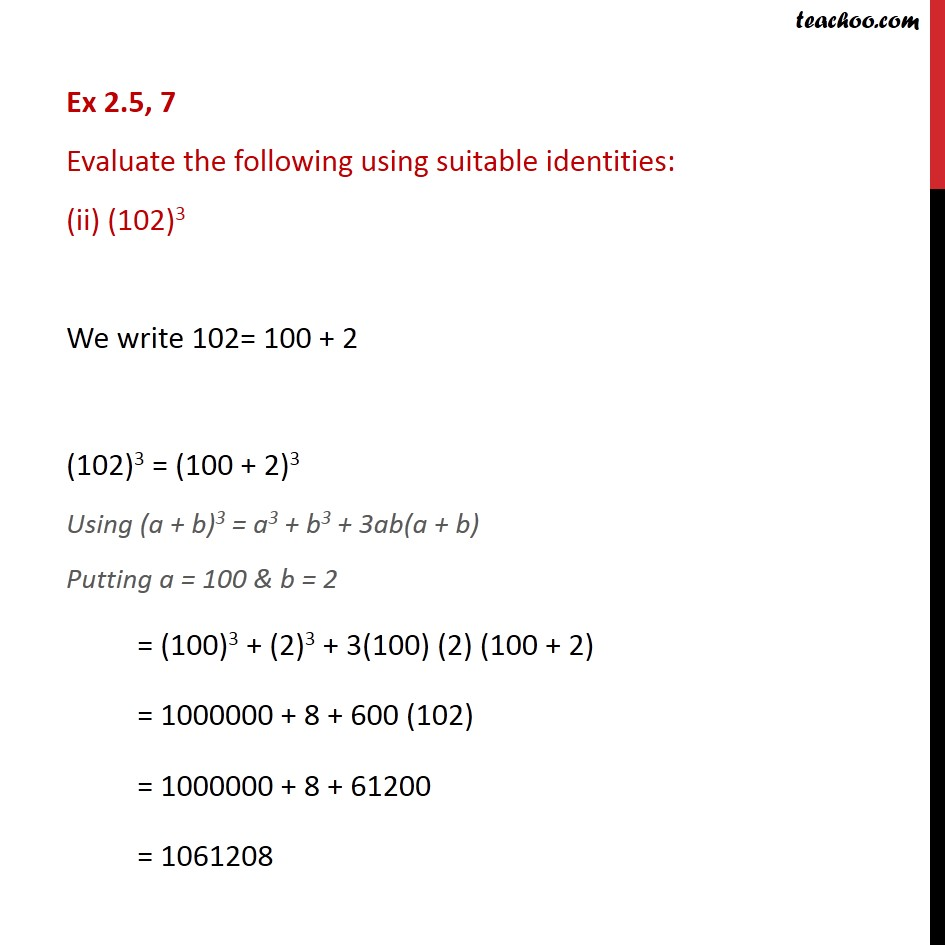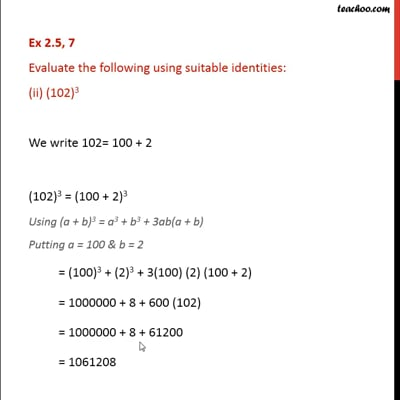Ex 2.5

Chapter 2 Class 9 Polynomials
Serial order wiseThis video is only available for Teachoo black users

Maths Crash Course - Live lectures + all videos + Real time Doubt solving!

### Transcript

Ex 2.5, 7 Evaluate the following using suitable identities: (ii) (102)3 We write 102= 100 + 2 (102)3 = (100 + 2)3 Using (a + b)3 = a3 + b3 + 3ab(a + b) Putting a = 100 & b = 2 = (100)3 + (2)3 + 3(100) (2) (100 + 2) = 1000000 + 8 + 600 (102) = 1000000 + 8 + 61200 = 1061208